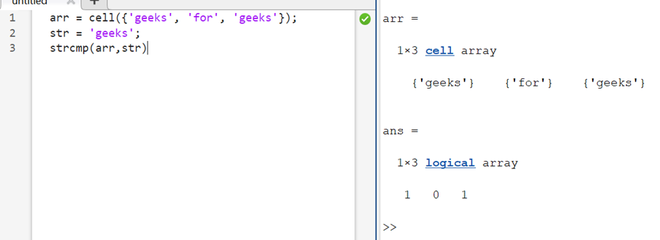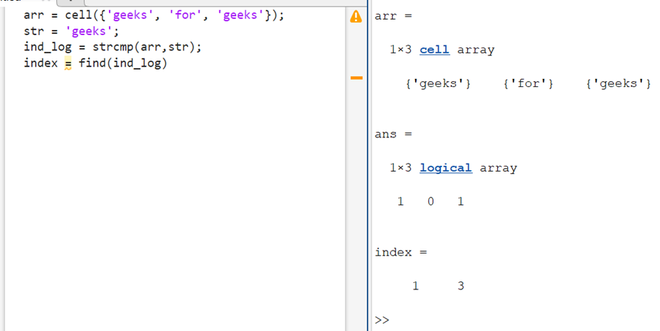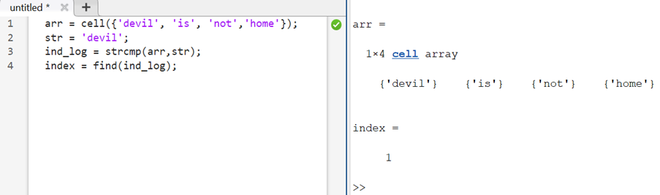Open in App
Not now

# MATLAB Find Exact String in Cell Array

• Last Updated : 22 Nov, 2022

Cell arrays in MATLAB store data of various data types as a cell. These cells could contain data of different types but belong to the same array. Now, this article is focused on finding an exact string in a cell array in MATLAB. This can be done easily by using a combination of two MATLAB functions, the strcmp() and find() functions. Let us see how the same is done

Syntax:

s_log = strcmp(<array>,<string>)

index = find(s_log)

This will return a vector with indices of all string elements that exactly match the given string. The strcmp() function takes an array and a string as input. Then it compares the string with all values in the passed array and returns a logical array with 1 on matched cells and 0 on unmatched ones. See below to understand the same.

Example 1:

## Matlab

 `% MATLAB program for find Exact String in Cell Array``% cell array``arr = cell({``'geeks'``, ``'for'``, ``'geeks'``});`` ` `% String to be checked``str = ``'geeks'``;    `` ` `% Getting  a logical array from strcmp``strcmp(arr,str)`

Output:

This should return a vector [1 0 1] as the string str appears twice in the array arr on indices 1 and 3.Now, we can use the find function to get the indices of non-zero elements from this logical array, which will be the same as the indices of appearance of our ‘str’ string.

Example 2:

## Matlab

 `% MATLAB program ``arr = cell({``'geeks'``, ``'for'``, ``'geeks'``});``str = ``'geeks'``;``ind_log = strcmp(arr,str);``index = find(ind_log)`

Output:As it can be verified, the string ‘geeks’ appear on two indices 1 and 3, and the same result is given by our program.  Let us take another example where we find the string ‘devil’ in a given cell array.

Example 3:

## Matlab

 `% MATLAB code for array``arr = cell({``'devil'``, ``'is'``, ``'not'``,``'home'``});`` ` `% String``str = ``'devil'``;`` ` `% Finding logical array``ind_log = strcmp(arr,str);`` ` `%Finding indices using find functions``index = find(ind_log);`

Output:My Personal Notes arrow_drop_up